August 29, 2017

## Learning Objectives

• How to install an R package.
• How to call an R package.
• How to plot data.
• Understand xts-objects
• Transform xts-objects to ts-objects
• Produce simple forecasts

## Installing and Loading R Packages

You might need to install an R package first

install.packages("pdfetch")

You need to call the library in the current session

library(pdfetch)

Now let us get some real data - the US unemployment rate in this case …

un.xts <- pdfetch_FRED("UNRATE")

## Required Libraries

Here is a couple of libraries that we will need going forward

library(pdfetch)
library(forecast)
library(seasonal)
library(fpp)
library(TSA)
library(xts)

Now let us get some real data

un.xts <- pdfetch_FRED("UNRATE")

## Plotting Data in xts

Now we got the data. Let us plot it first.

plot(un.xts)
## Loading required package: zoo
##
## Attaching package: 'zoo'
## The following objects are masked from 'package:base':
##
##     as.Date, as.Date.numeric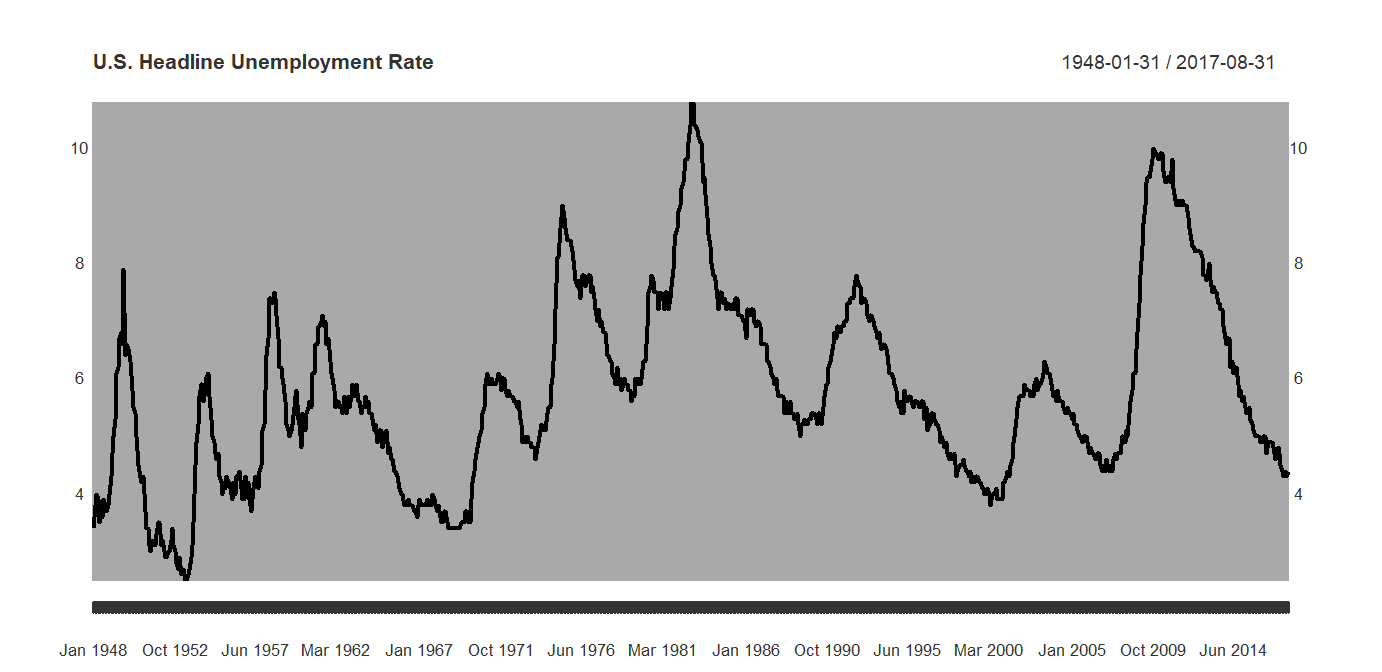## What is xts?

The unemployment rate is a so called xts object

str(un.xts)
## An 'xts' object on 1948-01-31/2017-08-31 containing:
##   Data: num [1:836, 1] 3.4 3.8 4 3.9 3.5 3.6 3.6 3.9 3.8 3.7 ...
##  - attr(*, "dimnames")=List of 2
##   ..$: NULL ## ..$ : chr "UNRATE"
##   Indexed by objects of class: [Date] TZ: UTC
##   xts Attributes:
##  NULL

## What is xts?

The unemployment rate is a so called xts object

class(un.xts)
##  "xts" "zoo"

There a lot of stuff you can do with xts, but for our purposes ts is perfectly fine. See here if you want to know more about xts features.

## Subsetting in xts

How did unemployment do since 2010?

plot(un.xts['2010/'])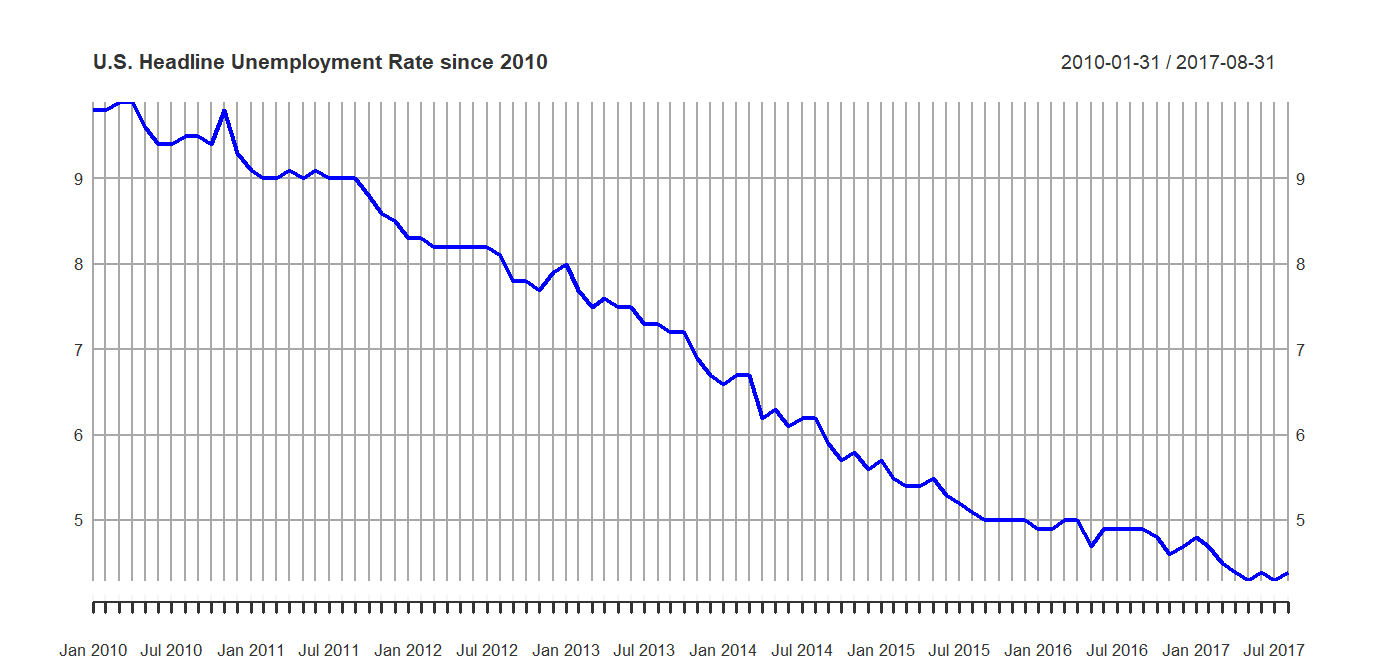## Subsetting in xts

How did unemployment do since before 1970?

plot(un.xts['/1970'])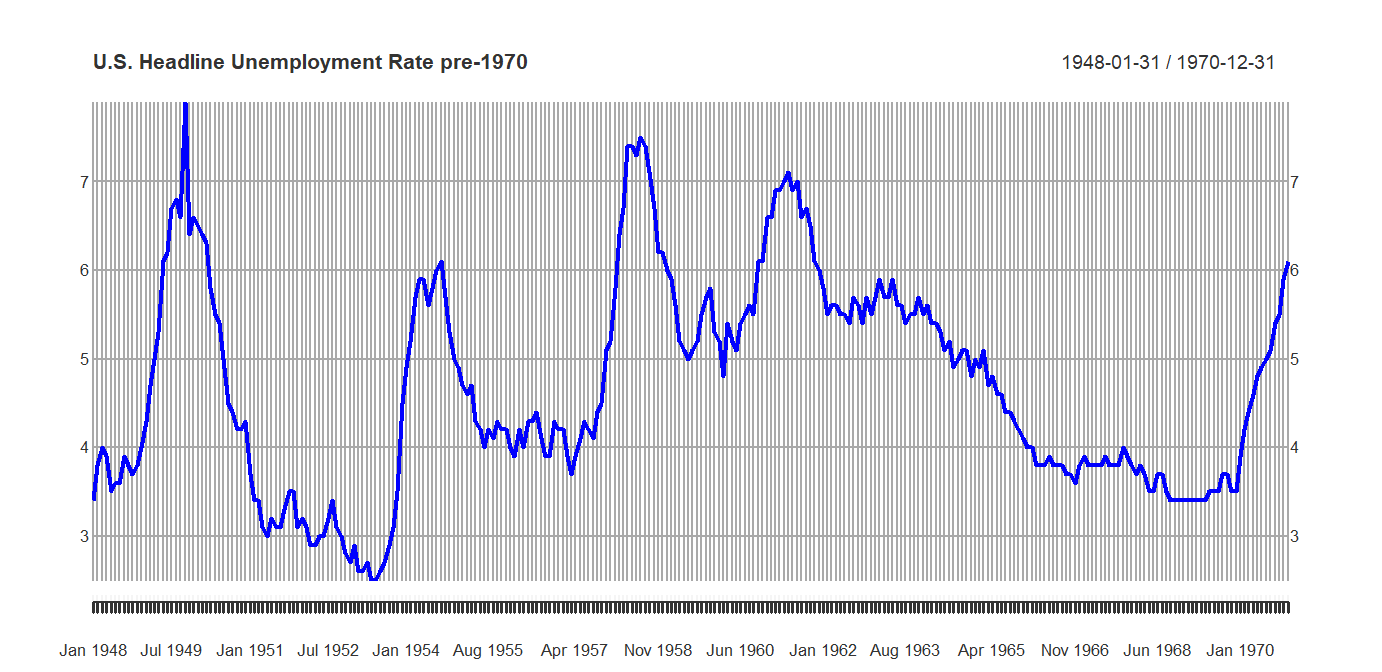## Subsetting in xts

How did unemployment do in the 1990s?

plot(un.xts['1990/2000'])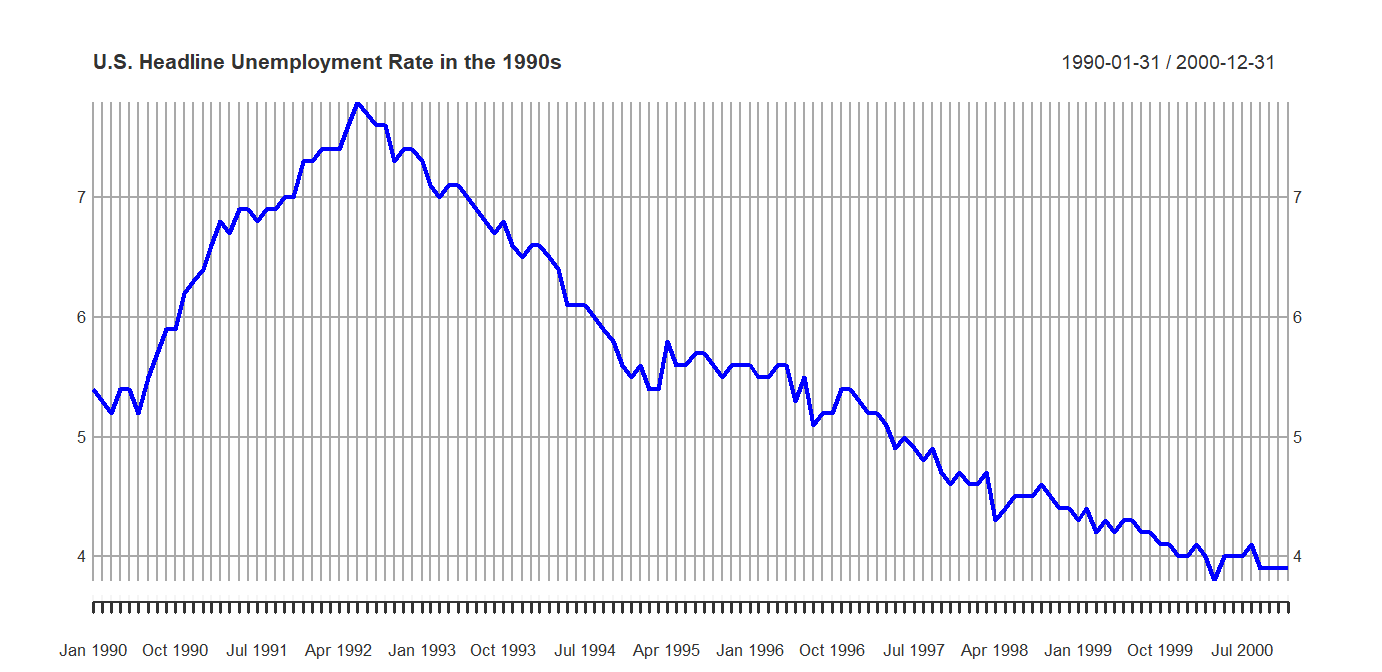## From xts to ts

start(un.xts)
##  "1948-01-31"
end(un.xts)
##  "2017-08-31"
un.ts = ts(un.xts, start = c(1947,1),
end = c(2017,7), frequency = 12)

un.ts is now a ts object that may be used with the forecast package.

## Plotting Data in ts

Now we got the data. Let us plot it first.

plot(un.ts)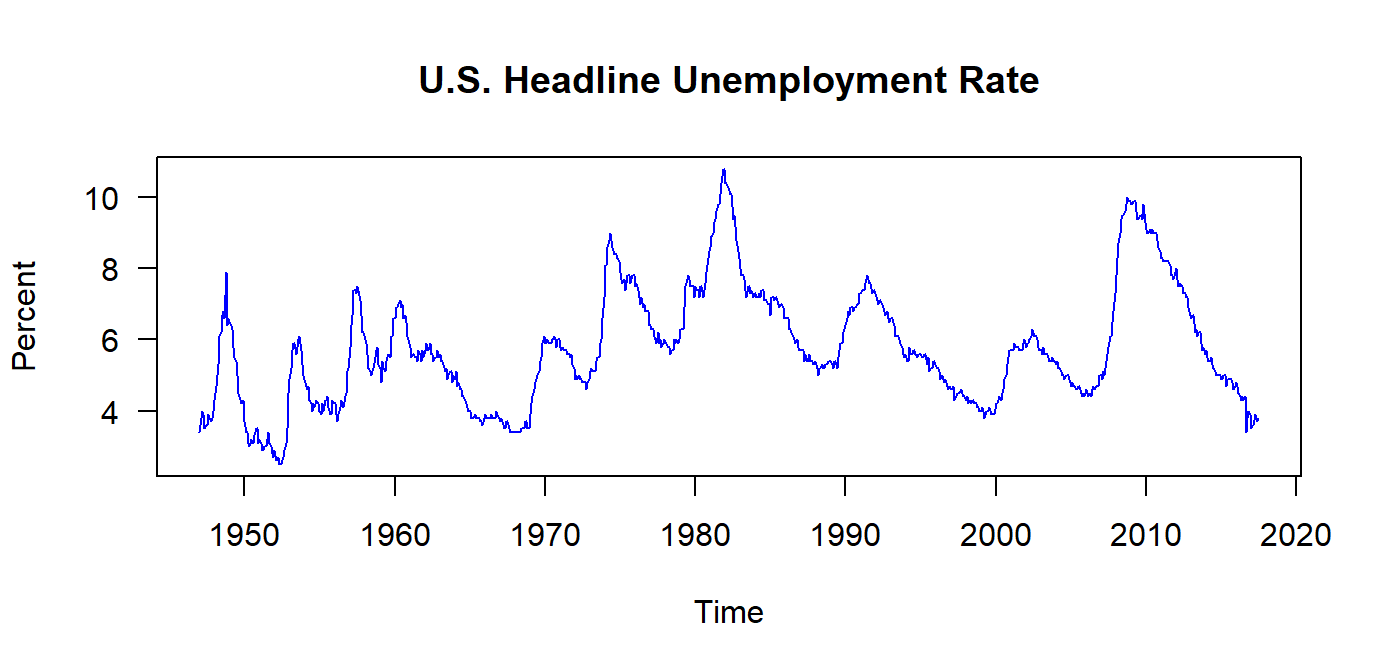## Subsetting in ts

How did unemployment do since 2010?

plot(window(un.ts, start = c(2000,1)))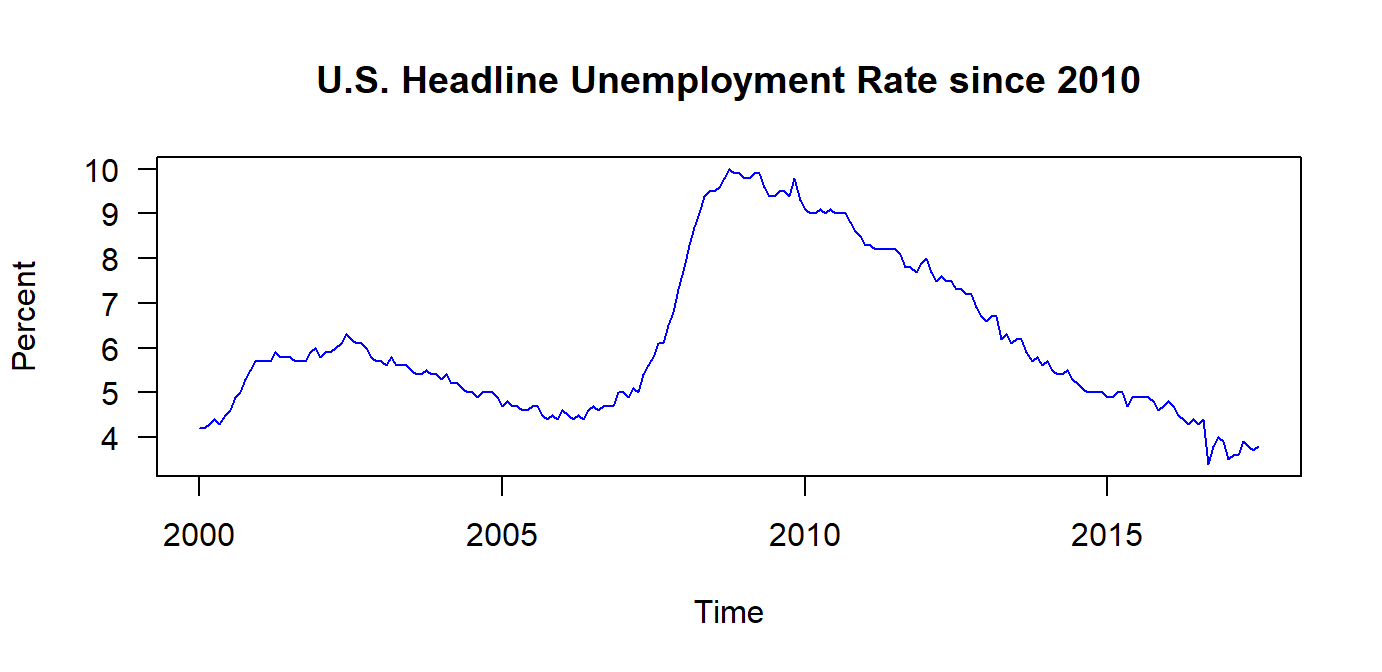## Subsetting in ts

How did unemployment do since before 1970?

plot(window(un.ts, end = c(1970,12)))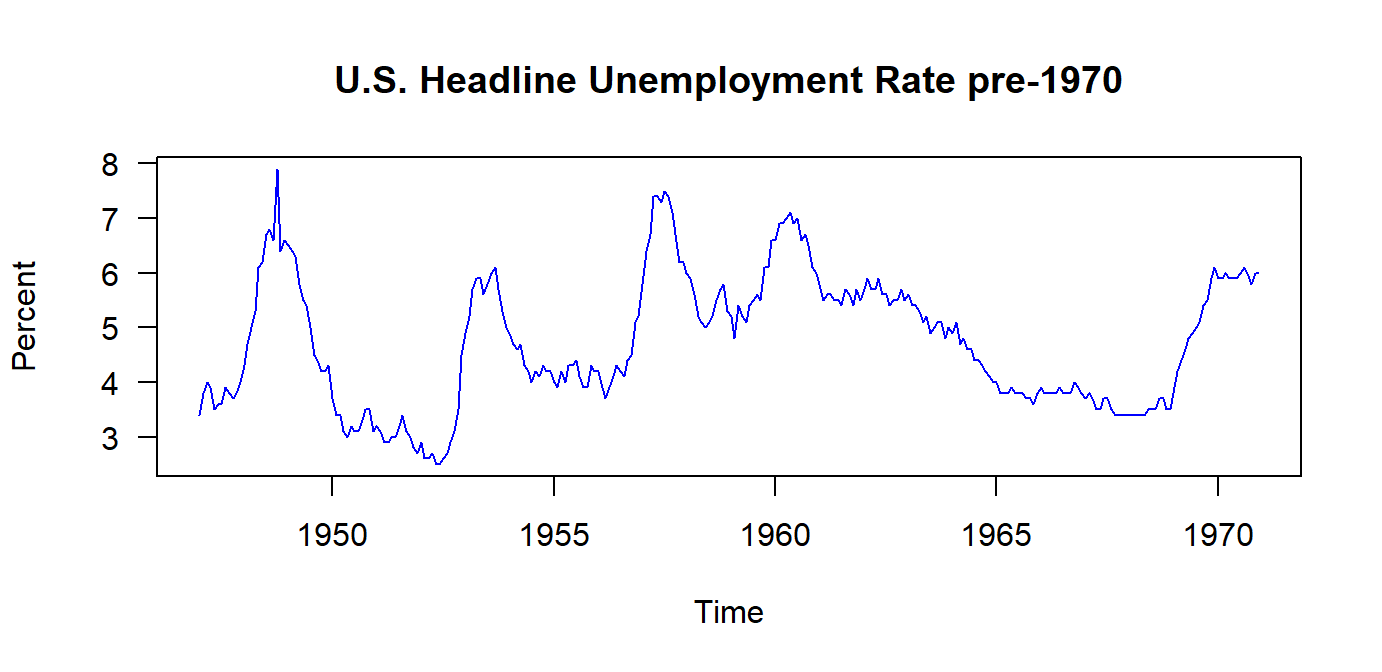## Subsetting in ts

How did unemployment do in the 1990s?

plot(window(un.ts, start = c(1990,1), end = c(1999,12)))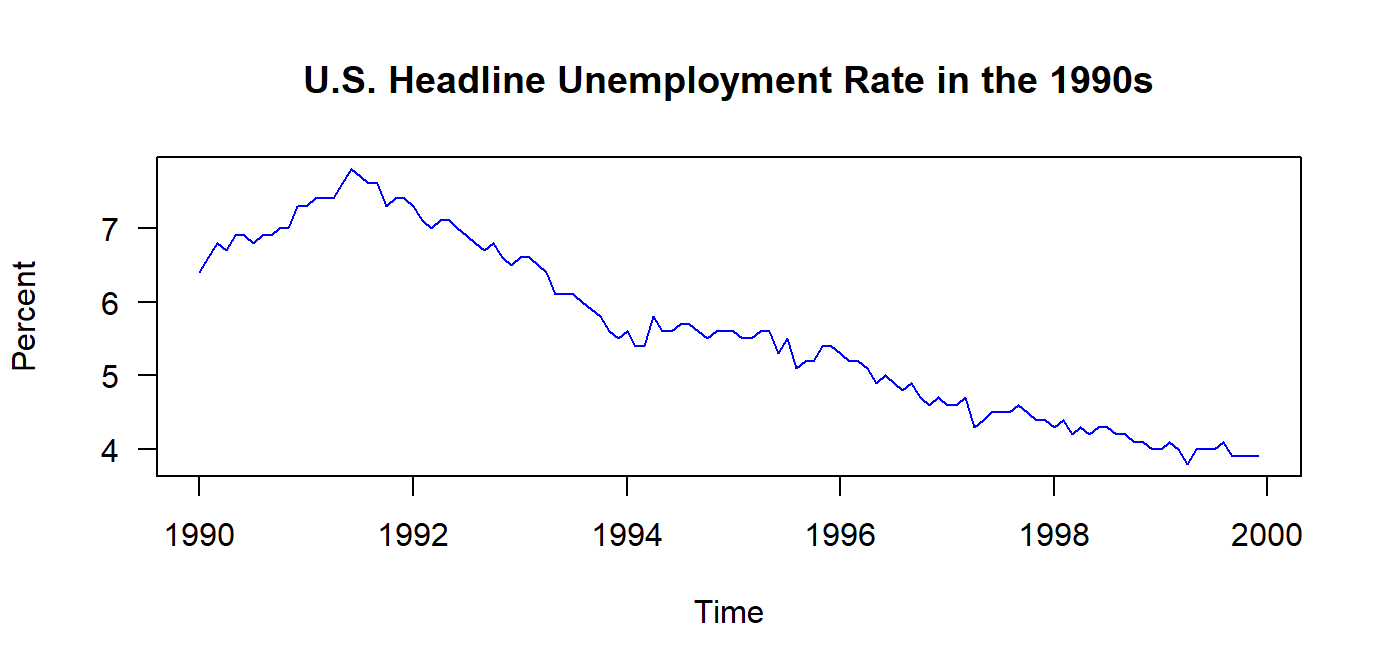## Why do we do all this "subsetting"?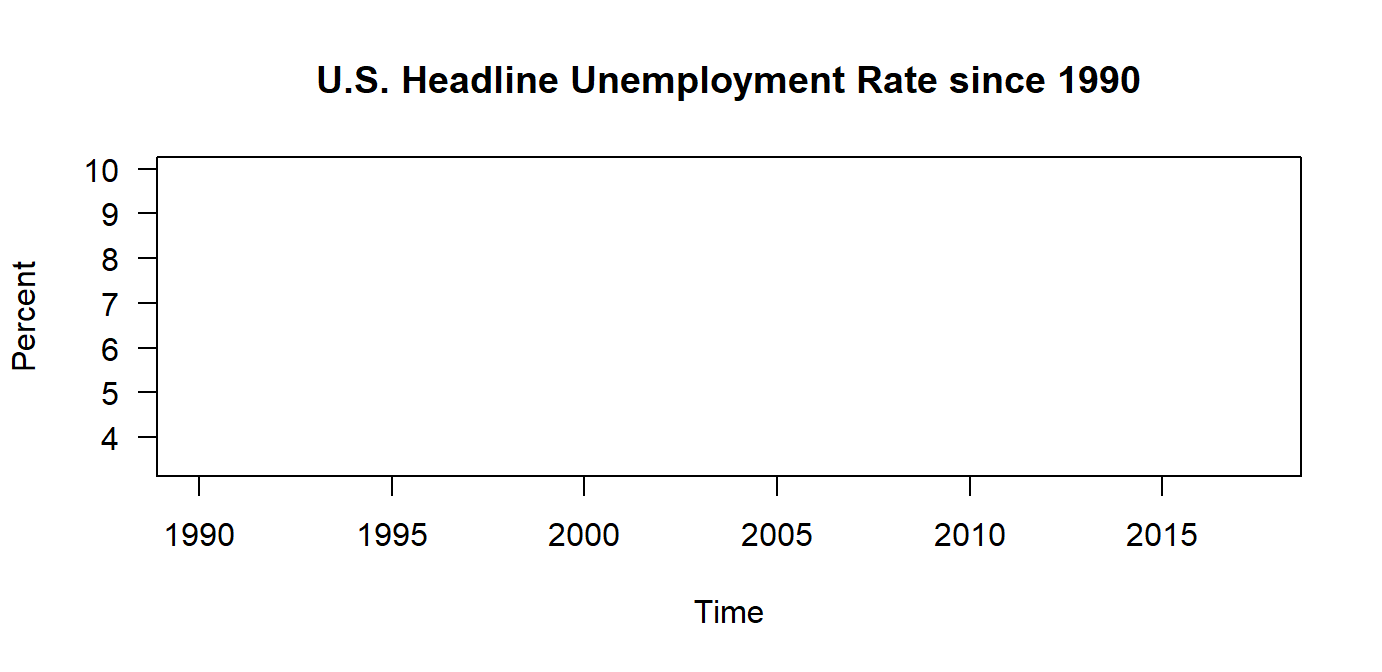## Why do we do all this "subsetting"?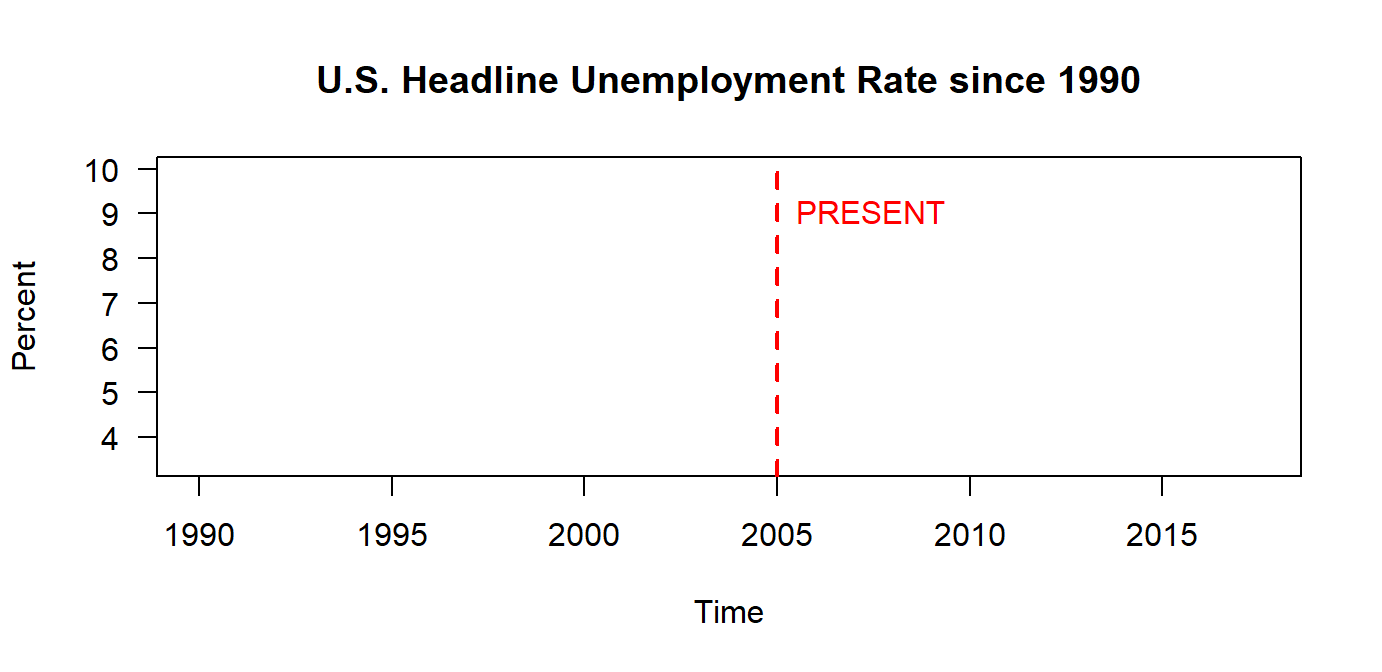## Why do we do all this "subsetting"?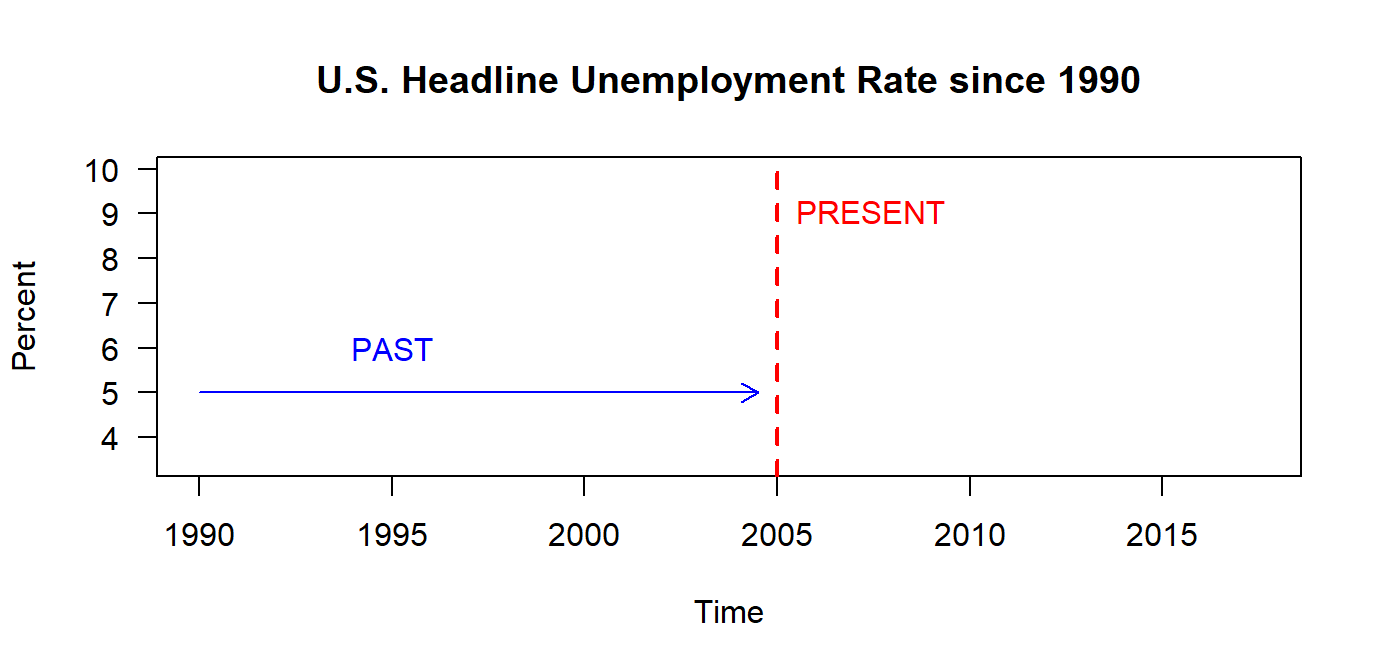## Why do we do all this "subsetting"?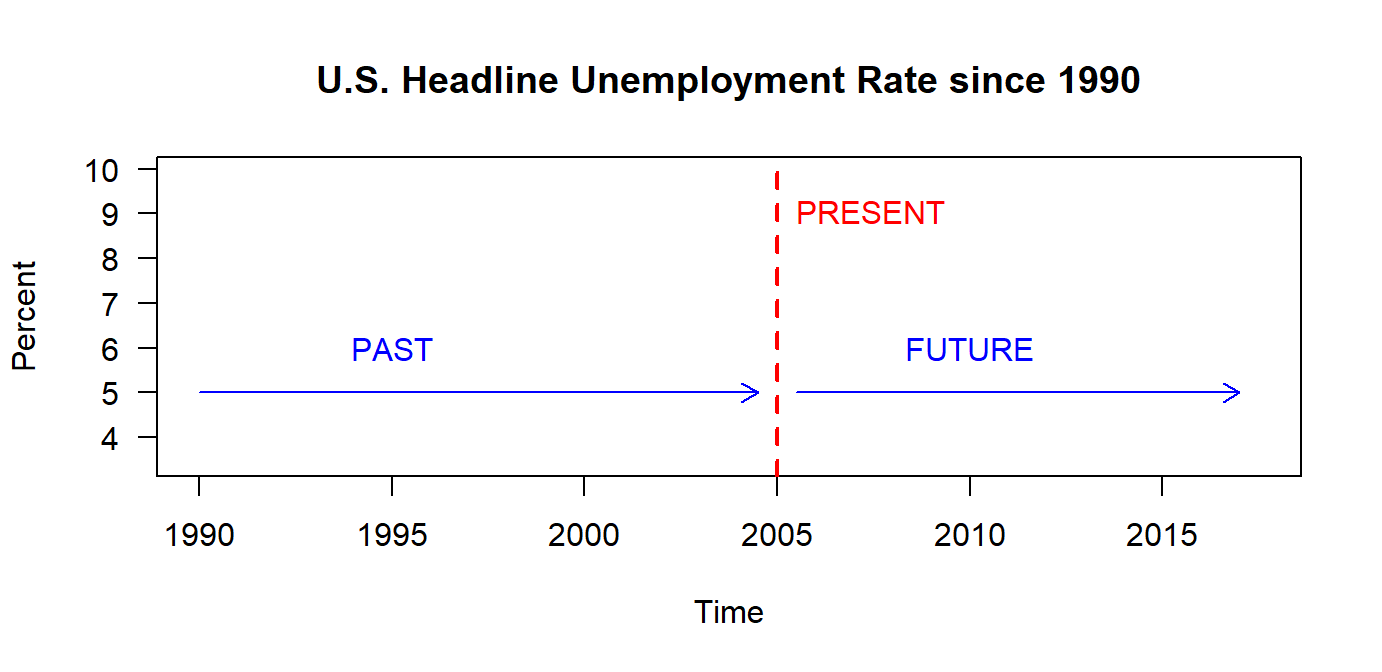## Why do we do all this "subsetting"?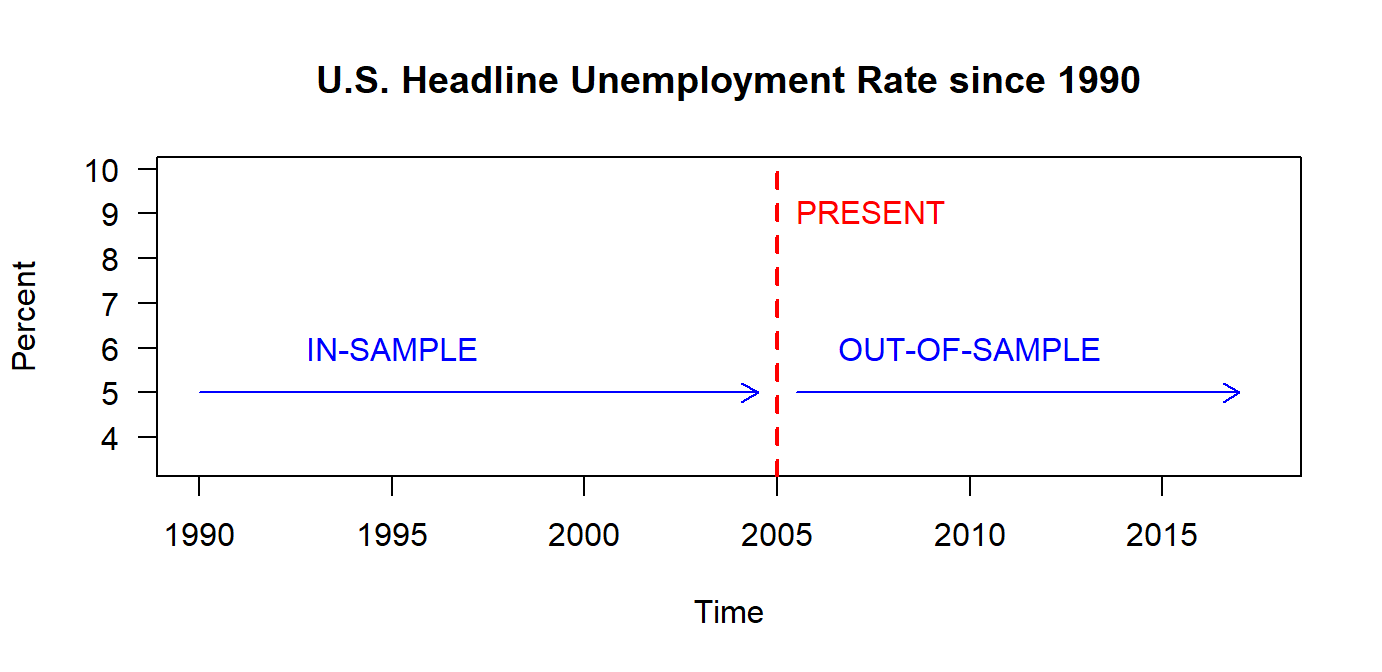## Why do we do all this "subsetting"?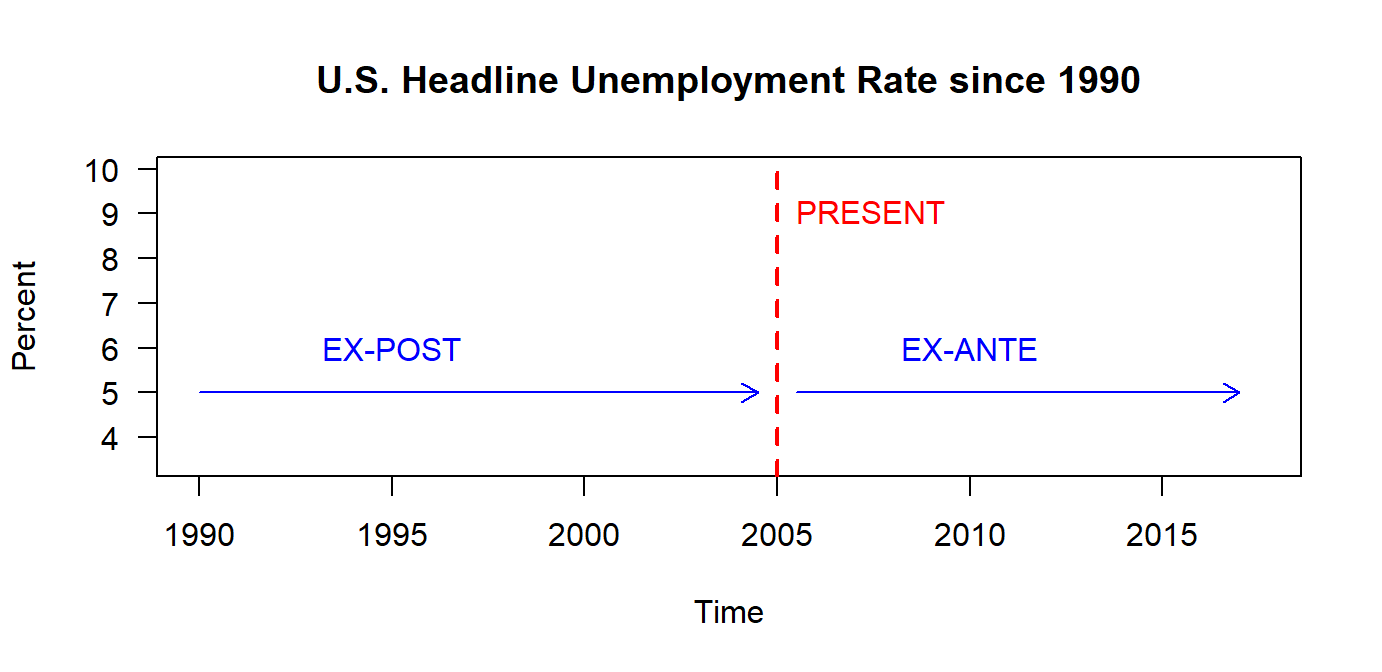## Simple Forecast – 1. Average Method

• Forecast of all future values is equal to mean of historical data $${y_1, \cdots, y_T}$$

• Forecast: $\hat{y}_{T+h|T}=\bar{y}=(y_1+ \cdots+y_T)/T$

## Simple Forecast – 1. Average Method

un.f.meanf <- meanf(un.ts,h=60)
plot(un.f.meanf,las=1,col="blue",ylim=c(-2,10))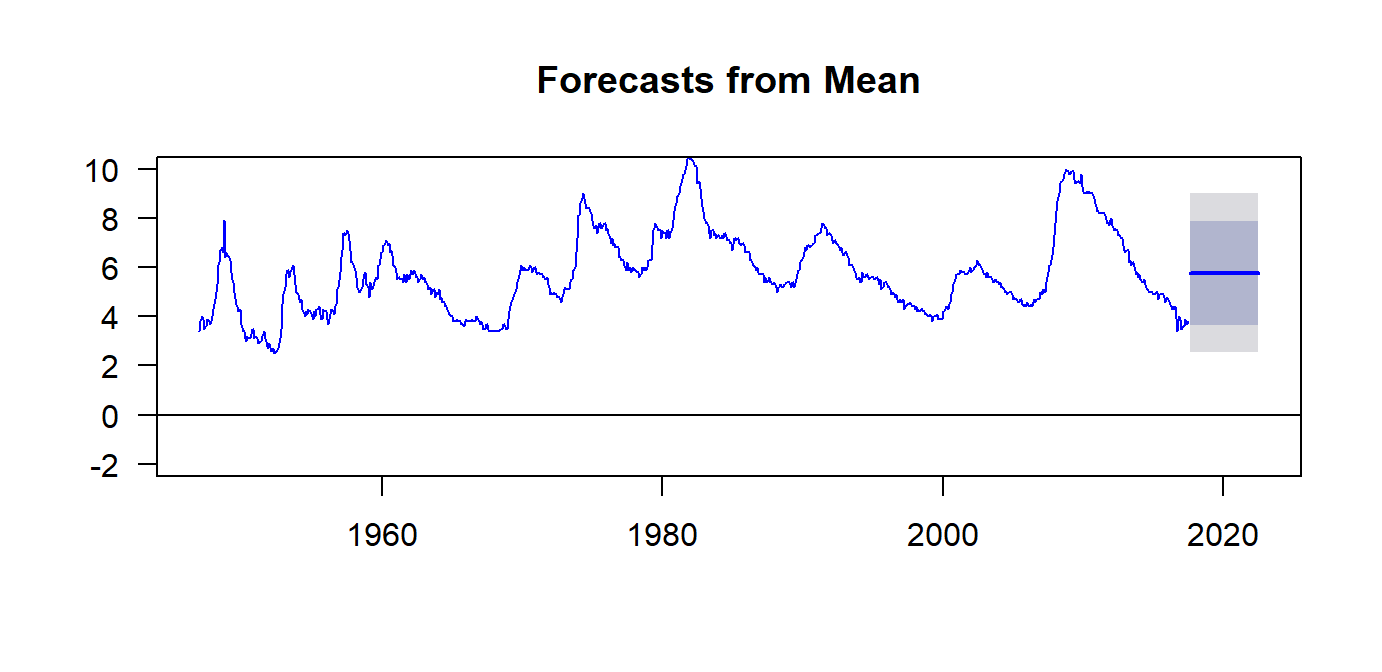## Simple Forecast – 2. Naïve

• Forecast equal to last observed value

• Forecast: $\hat{y}_{T+h|T} = y_{T|T}$
• Optimal for efficient stock markets.

## Simple Forecast - 2. Naïve

un.f.naive <- naive(un.ts,h=60)
plot(un.f.naive,las=1,col="blue",ylim=c(-2,10))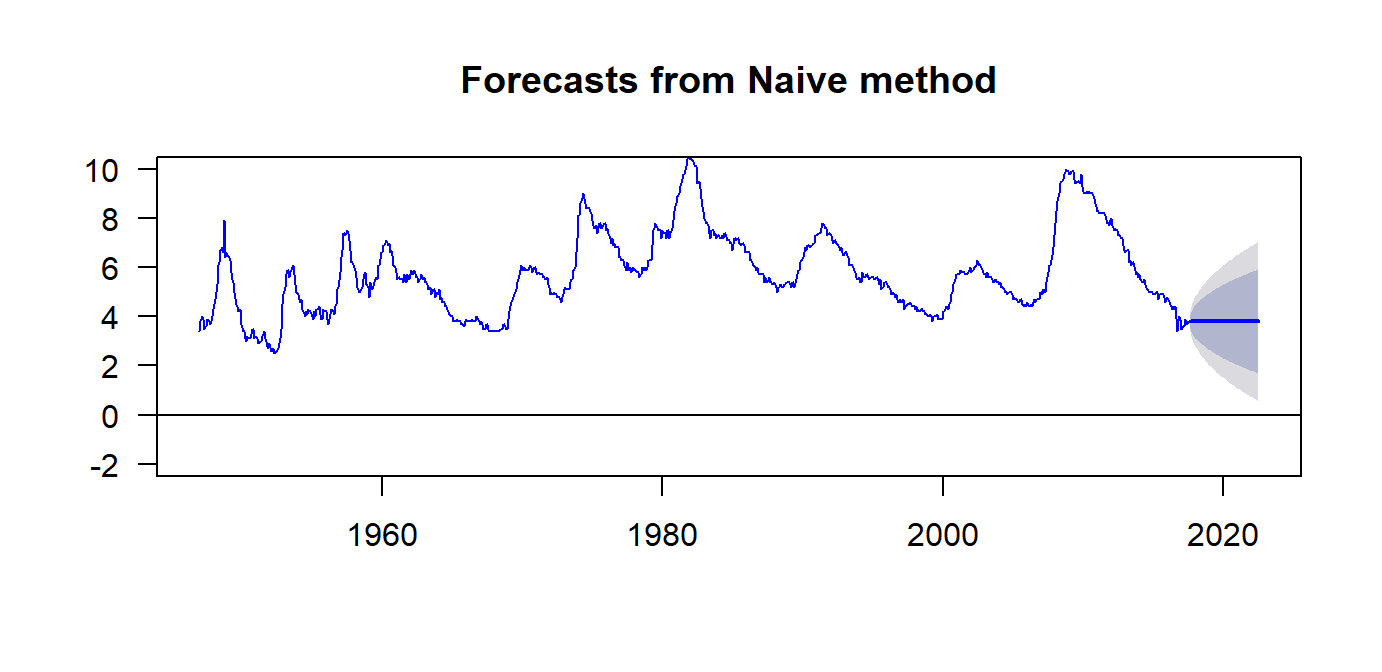## Simple Forecast – 3. Seasonally Naïve

• Forecast equal to last value from same season

• Forecast: $y_{T+h-km}$ where $$m=$$ seasonal period, $k=\lfloor (h-1)/m\rfloor+1$

## Simple Forecast – 3. Seasonally Naïve

un.f.snaive <- snaive(un.ts,h=60)
plot(un.f.snaive,las=1,col="blue",ylim=c(-2,10))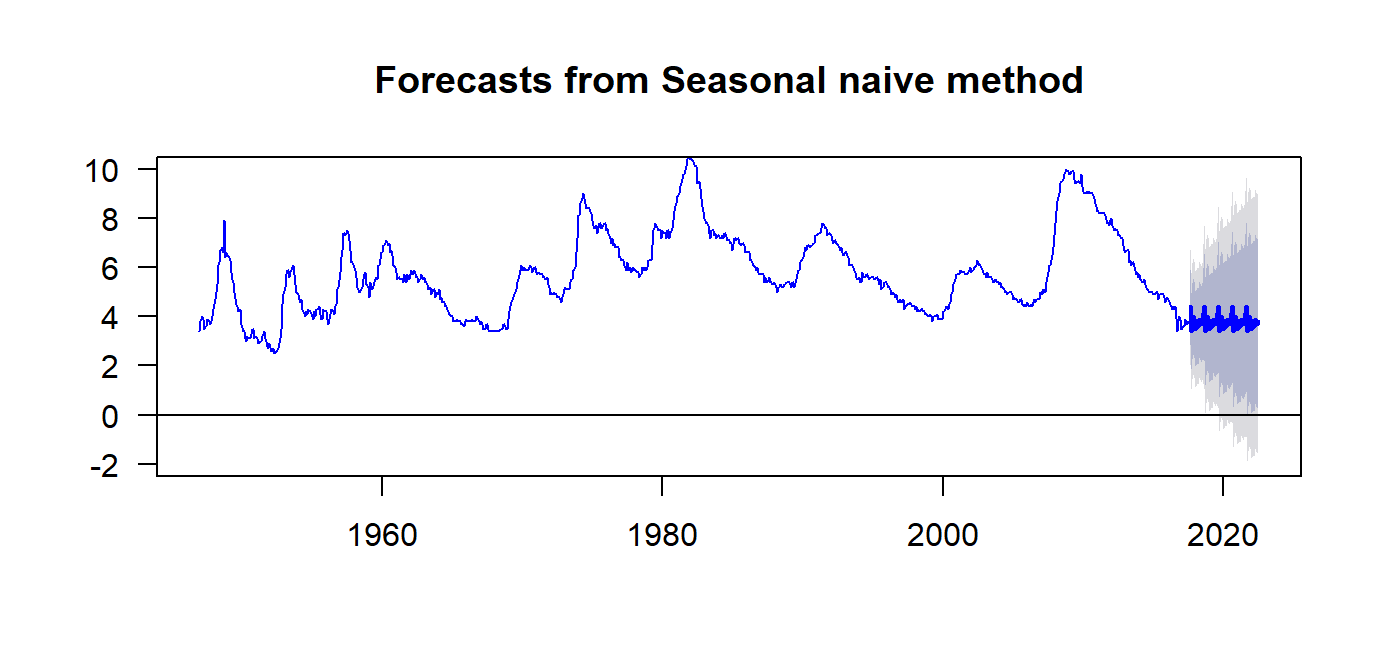## Simple Forecast – Forecast Objects

un.f.meanf <- meanf(un.ts,h=60)
un.f.naive <- naive(un.ts,h=60)
un.f.snaive <- snaive(un.ts,h=60)
class(un.f.meanf)
##  "forecast"
class(un.f.naive)
##  "forecast"
class(un.f.snaive)
##  "forecast"

## What is in the Forecast Object?

str(un.f.meanf)
head(un.f.meanf$lower,3) ## 80% 95% ## Aug 2017 3.664616 2.547679 ## Sep 2017 3.664616 2.547679 ## Oct 2017 3.664616 2.547679 un.f.meanf$method
un.f.meanf$level ## What is in the Forecast Object? un.f.meanf$x
un.f.meanf$series un.f.meanf$mean
un.f.meanf$lower un.f.meanf$upper
AP.meanf$model un.f.meanf$fitted
un.f.meanf$residuals ## Residuals For this particular model: Why do the residuals look the way they look? plot(un.f.meanf$residuals, col = "blue", ylim = c(-4,12))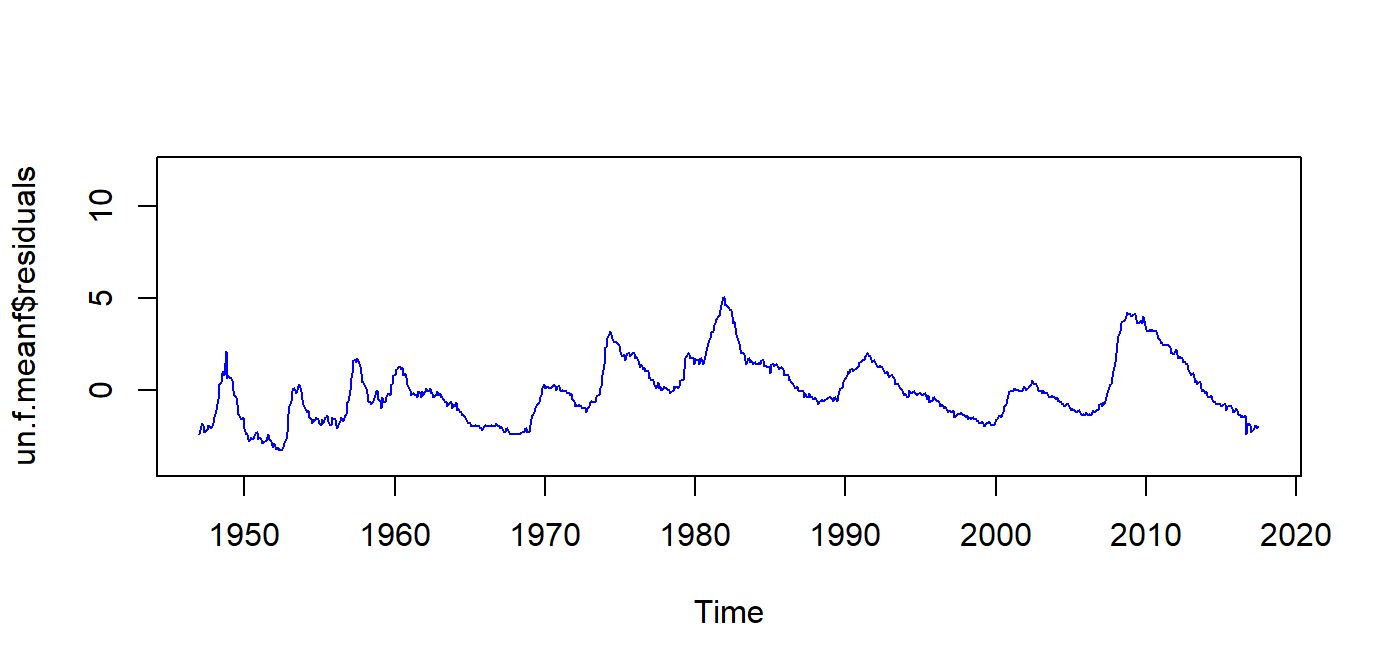## Residuals

For this particular model: Why do the residuals look the way they look?

abline(h=0, col = "black")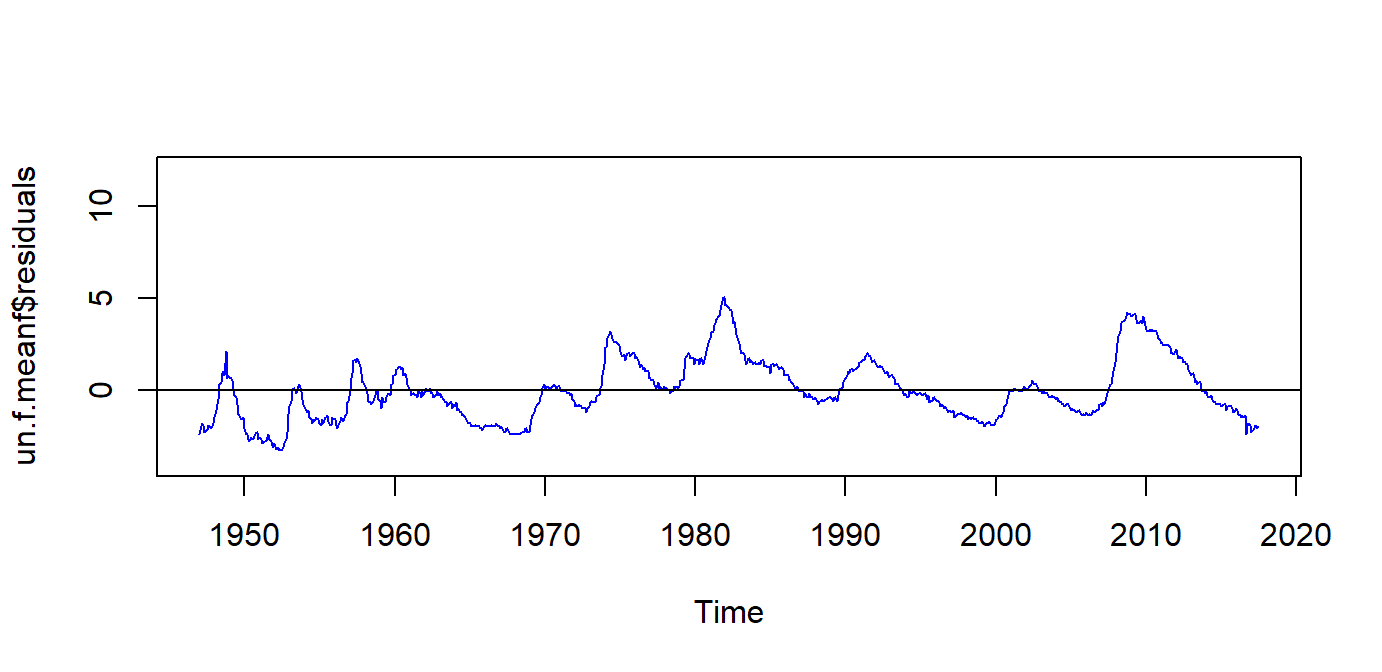## Residuals

For this particular model: Why do the residuals look the way they look?

lines(un.ts, col = "red")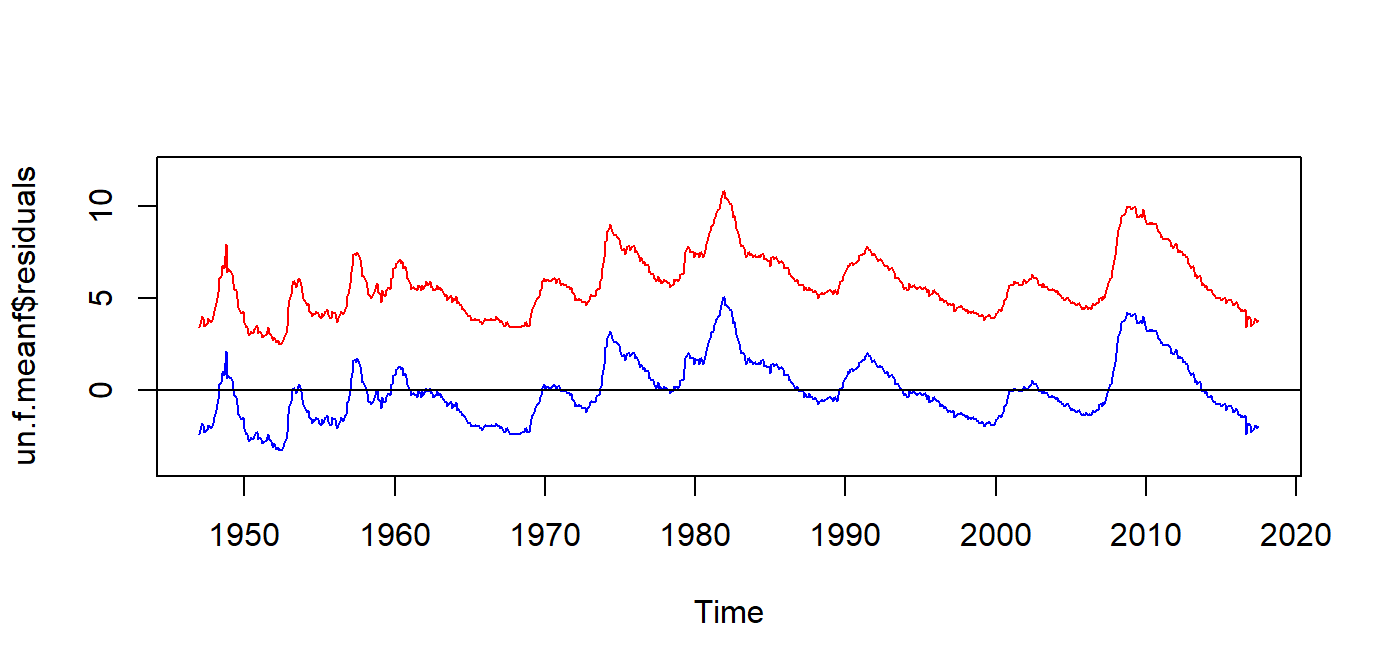## What have we learned today?

4. Transforming xts to ts
5. Some simple forecasting methods using forecast package